# What Are The Zeros Of A Function? (5 Key Ideas)

A zero of a function can help us to solve an equation.  It can also help with optimization problems (such as when we find the zeros of the first or second derivative of a function).

So, what are the zeros of a function?  A zero of a function is an input (x-value) that yields an output (y-value) of zero. On a graph, a zero of a function is where the curve intersects the line y = 0 (the x-axis). A function can have 0, 1, 2, or more zeros, depending on the type of function. Some functions have infinitely many zeros!

Of course, a polynomial of degree n has at most n distinct real roots (and exactly n complex roots) by the Fundamental Theorem of Algebra.

In this article, we’ll talk about zeros of functions and how they look on a graph.  We’ll also look at how to find the zeros of a function and ways to tell how many zeros a function can have.

Let’s get started.

## What Are The Zeros Of A Function?

The zeros of a function are all the inputs (values of x) that lead to an output (y-value) of zero.  If we look at the graph of a function, a zero is a place where the function intersects the line y = 0 (the x-axis).

Note that a function can have no zeros at all if it never intersects the x-axis.  (Note: the function may still have complex zeros, even if it has no real zeros).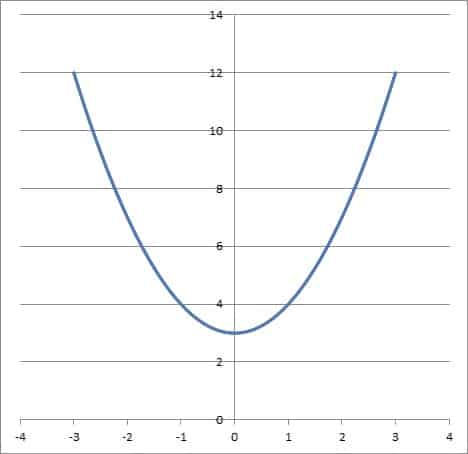The function f(x) = x2 + 3 has no real zeros, since it does not intersect the line y = 0 (the x-axis).

A function can also have 1, 2, 3, and more zeros (depending on the type of function).

In some cases, it is even possible for a function to have infinitely many zeros (for example, the sine function – more on this later!)

Note that any function with more than one zero is not a one-to-one function.  This is due to the fact that the graph will fail the horizontal line test for the line y = 0 (that is, the function intersects the x-axis more than once).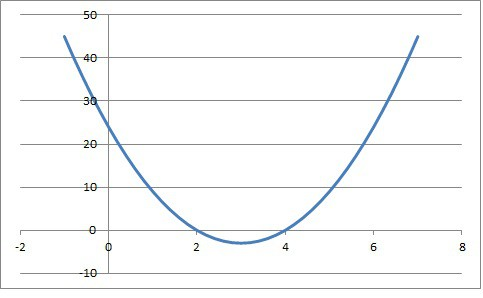The function f(x) = 3x2 -18x + 24 has two real zeros at x = 2 and x = 4. As a result, it is not one-to-one (it fails the horizontal line test for y = 0).

## What Are The Zeros Of A Function Called?

The zeros of a function are also called roots.  These are values of x (inputs) that make the function equal to zero (an output of zero, or y = 0).

If we have a function f(x), the zeros (or roots) of f(x) are also called solutions to the equation f(x) = 0.

Remember that for a zero “c” of a function f(x), the ordered pair will be (c, 0).

## What Is A Zero On A Graph?

A zero on a graph is a point where the function y = f(x) intersects the line y = 0 (the x-axis).  These points also indicate solutions to the equation f(x) = 0.

You can find the zeros of a function from a graph by visual inspection.

### Example 1: Finding The Zeros Of A Function From Its Graph

Consider the function f(x) = x2 – 5x + 4.  Its graph is shown below: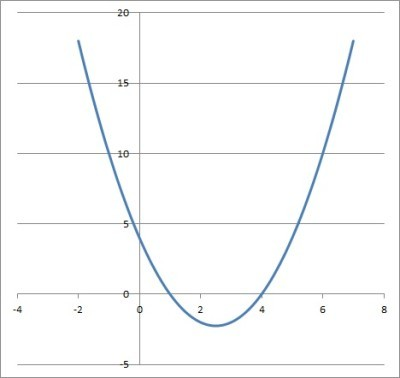The function f(x) = x2 – 5x + 4 has zeros at x = 1 and x = 4.

By inspecting the graph, we can see that the function intersects the x-axis at x = 1 and x = 4.  This means that the zeros of f(x) are x = 1 and x = 4.

We can also say that f(1) = 0 and f(4) = 0.

Now that we know the roots of the function, we can factor it easily:

• f(x) = x2 – 5x + 4
• f(x) = (x – 1)(x – 4)

### Example 2: Finding The Zeros Of A Function From Its Graph

Consider the function f(x) = x3 – 3x2 + 2x.  Its graph is shown below: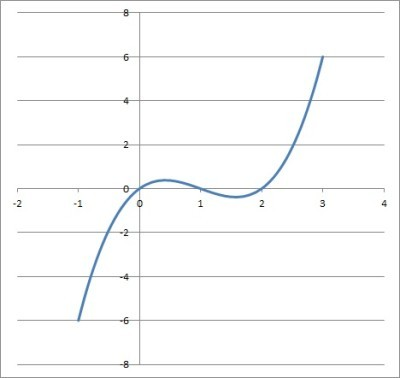The function f(x) = x3 – 3x2 + 2x has zeros at x = 0, x = 1, and x = 2.

By inspecting the graph, we can see that the function intersects the x-axis at x = 0, x = 1, and x = 2.  This means that the zeros of f(x) are x = 0, x = 1, and x = 2.

We can also say that f(0) = 0, f(1) = 0, and f(2) = 0.

Now that we know the roots of the function, we can factor it easily:

• f(x) = x3 – 3x2 + 2x
• f(x) = (x – 0)(x – 1)(x – 2)
• f(x) = x(x – 1)(x – 2)

### Example 3: Finding The Zeros Of A Function From Its Graph

Consider the function f(x) = x2 + 4.  Its graph is shown below: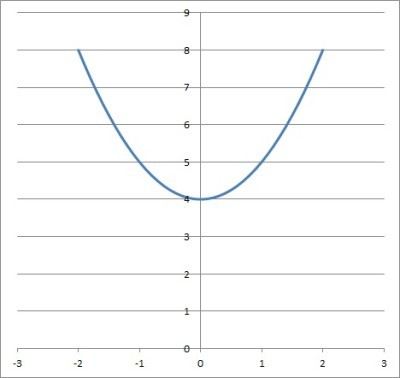The function f(x) = x2 + 4 has no real zeros, since it never intersects the line y = 0 (the x-axis).

By inspecting the graph, we can see that the function never intersects the x-axis.  This means that the function f(x) has no real zeros (its zeros are complex numbers with nonzero imaginary parts).

It turns out that the zeros are pure imaginary numbers: x = 2i and x = -2i (they are complex conjugates).  We can find them by solving the equation:

• x2 + 4 = 0
• x2 = -4
• x = √(-4)
• x = 2i or x = -2i

## How Many Zeros Does A Function Have?

A function can have any number of zeros, depending on the type of function.  A function can have 0, 1, 2, 3, or more zeros.

A function can also have infinitely many zeros!

The Fundamental Theorem of Algebra tells us that:

• A polynomial of degree n in one variable with complex coefficients has n complex roots.

So a linear function (with degree n = 1) can have only one complex root.

The table below compares some functions and the number of zeros they have.

### Can A Quadratic Function Have More Than Two Zeros?

A quadratic function cannot have more than two zeros.  By the Fundamental Theorem of Algebra, a quadratic function (which has degree n = 2) has only two complex zeros.

However, a quadratic function need not have two real roots.  It could have one real root (a double root or repeated root) or no real roots (in the case of two complex conjugate roots with nonzero imaginary parts).

For a quadratic function f(x) = ax2 + bx + c, these cases are entirely determined by the discriminant D = b2 – 4ac of a quadratic as follows:

### Can A Cubic Function Have Two Zeros?

A cubic function cannot have exactly two real zeros (unless one of them is a double root).  By the Fundamental Theorem of Algebra, a cubic function (which has degree n = 3) has only three complex zeros.

By the Complex Conjugate Root Theorem, we know that complex roots come in pairs.  This tells us that a cubic function can have either 0 or 2 complex zeros with nonzero imaginary parts.

This, in turn, tells us that a cubic function can have 3 or 1 real zeros.

## How Do You Find The Zeros Of A Function?

To find the zeros of a function f(x), you must solve the equation f(x) = 0.  Sometimes this is not so easy!

Let’s look at some examples to see how it is done.

### Example 1: Finding The Zeros Of The Function f(x) = 2x + 6

Consider the linear function f(x) = 2x + 6.  Since it has degree n = 1, we know that there is only 1 root.

Setting the function equal to zero and solving gives us:

• f(x) = 0
• 2x + 6 = 0
• 2x = -6
• x = -3

So, x = -3 is the only zero of the function f(x) = 2x + 6.  You can verify this in the graph below.

### Example 2: Finding The Zeros Of The Function f(x) = x2 + 8x + 12

Consider the quadratic function f(x) = x2 + 8x + 12.  Since it has degree n = 2, we know that there are only 2 roots.

Setting the function equal to zero and solving gives us:

• f(x) = 0
• x2 + 8x + 12 = 0
• (x + 2)(x + 6) = 0
• x = -2, x = -6

So, x = -2 and x = -6 are the zeros of the function f(x) = x2 + 8x + 12.  You can verify this in the graph below.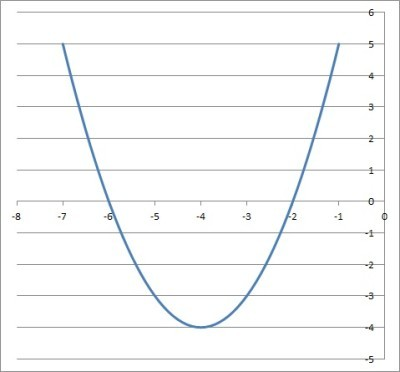The function f(x) = x2 + 8x + 12 has two real zeros, at x = -2 and x = -6.

### Example 3: Finding The Zeros Of The Function f(x) = x3 – 6x2  + 11x – 6

Consider the cubic function f(x) = x3 – 6x2  + 11x – 6.  Since it has degree n = 3, we know that there are only 3 roots.

Setting the function equal to zero and solving gives us:

• f(x) = 0
• x3 – 6x2  + 11x – 6 = 0
• (x – 1)(x – 2)(x – 3) = 0
• x = 1, 2, 3

So, x = 1, x = 2, and x = 3 are the zeros of the function f(x) = x3 – 6x2  + 11x – 6.  You can verify this in the graph below.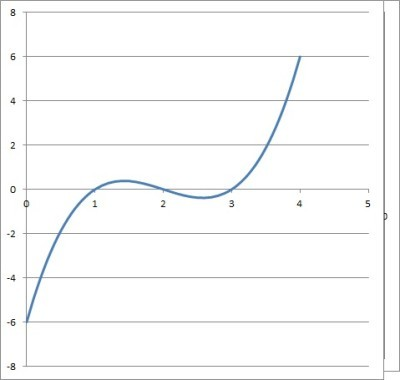The function f(x) = x3 – 6x2  + 11x – 6 has three real zeros: at x = 1, x = 2, and x = 3.

Since this function was not easy to factor, we could also graph the function first and find one or more zeros to make it easier to factor.

### Example 4: Finding The Zeros Of The Function f(x) = 2

Consider the function f(x) = 2.  Since the output (y-value) is always 2, regardless of the input (x-value), this function has no zeros.

You can verify this in the graph below.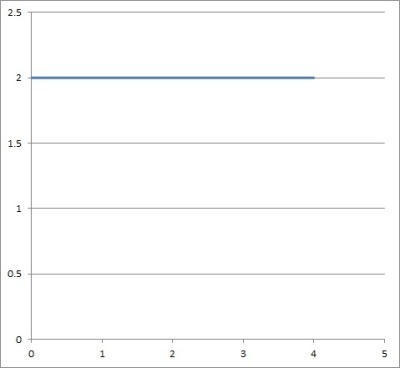The graph of the function f(x) = 2 has no zeros, since it never intersects the line y = 0 (the x-axis).

### Example 5: Finding The Zeros Of The Function f(x) = sin(x)

Consider the trigonometric function f(x) = sin(x).  Since it is not a polynomial function, we cannot use the Fundamental Theorem of Algebra to tell us how many roots there are.

Setting the function equal to zero and solving gives us:

• f(x) = 0
• sin(x) = 0

This equation is true for any integer multiple of π:

• x = …, -4π, -3π, -2π, -π, 0, π, 2π, 3π, 4π, …

This means that the function f(x) = sin(x) has infinitely many zeros on the domain of all real numbers.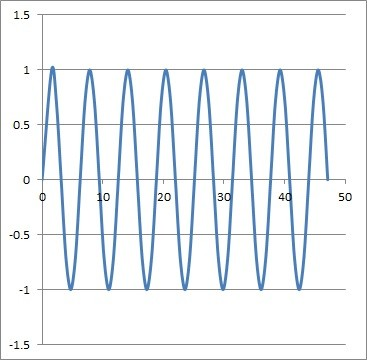The function f(x) = sin(x) has infinitely many zeros when its domain is the set of real numbers. Any integer multiple of pi is a zero of this function.

## Conclusion

Now you know what the zeros of a function are and how to find them.  You also know what they look like on a graph.